# Multiplicity dependence of two-particle azimuthal correlations in pp collisions at the LHC

We present the measurements of particle pair yields per trigger particle obtained from di-hadron azimuthal correlations in pp collisions at $\sqrt{s} = 0.9$, $2.76$, and $7$ TeV recorded with the ALICE detector. The yields are studied as a function of the charged particle multiplicity. Taken together with the single particle yields the pair yields provide information about parton fragmentation at low transverse momenta, as well as on the contribution of multiple parton interactions to particle production. Data are compared to calculations using the PYTHIA6, PYTHIA8, and PHOJET event generators.

Figures

## Figure 1

 Top panel: illustration of the contributions to the per-trigger pair yield as a function of $\dphi$. Bottom panel: the per-trigger pair yield as a function of $\dphi$ described by the fit function and its sub-components (see text).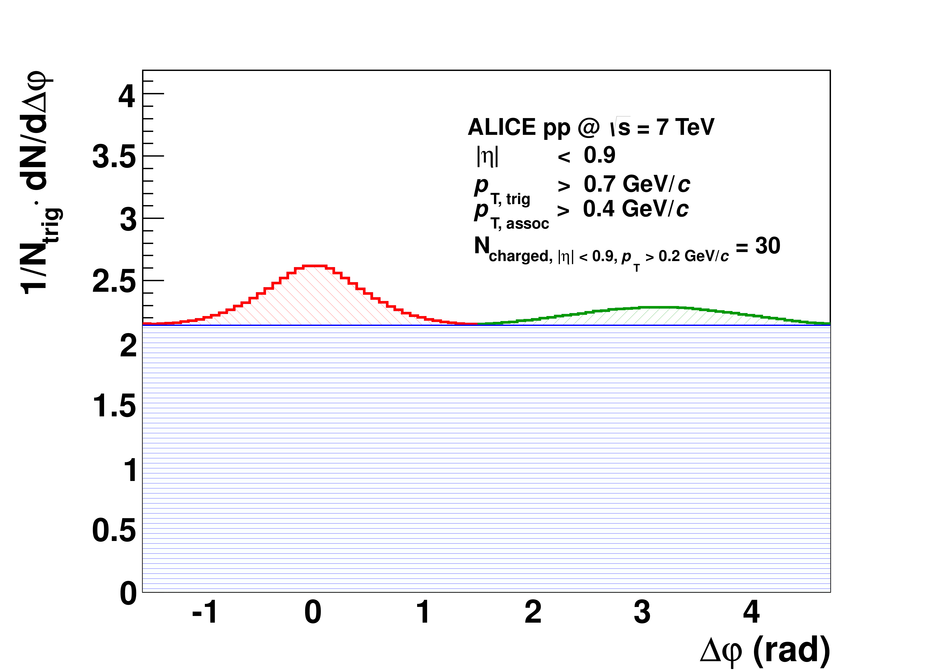## Figure 2

 Number of multiple parton interactions $N_{\rm MPI}$ in PYTHIA6 tune Perugia-0 and tune Pro-Q2O.## Figure 3

 Linear dependence between $N_{\rm uncorrelated\ seeds}$ and $N_{\rm MPI}$ in PYTHIA6 Perugia-0 simulations on generator level. Top panel: $N_{\rm uncorrelated\ seeds}$ versus $N_{\rm MPI}$ for different $|\eta|$-ranges. Bottom panel: $N_{\rm uncorrelated\ seeds}$ versus $N_{\rm MPI}$ for different $\pT$-trig thresholds.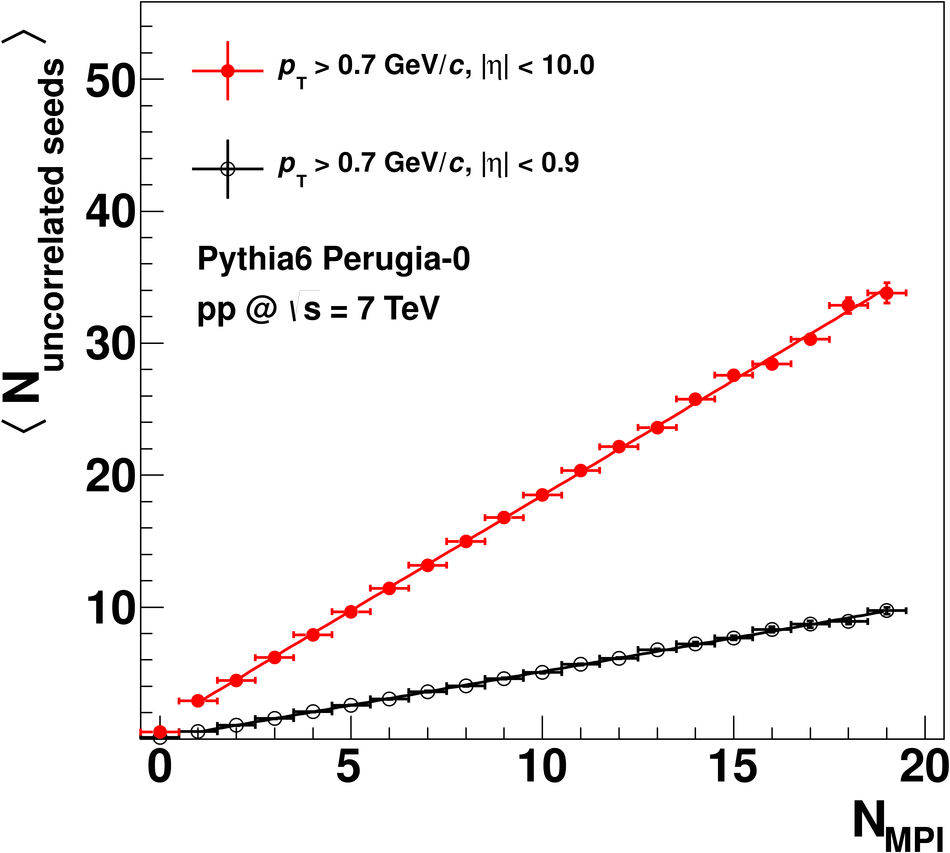## Figure 4

 Comparison of PYTHIA6 tunes Perugia-0 and Pro-Q2O for near-side pair-yield per trigger particle (top panel) and number of uncorrelated seeds (bottom panel).## Figure 5

 Reconstruction efficiency for primary particles. Top panel: reconstruction efficiency versus transverse momentum ($|\eta|< 0.9$). Bottom panel: reconstruction efficiency versus pseudorapidity ($p_{\mathrm{T}}>0.2$ GeV/$c$).## Figure 6

 Contamination correction.Top panel: contamination correction versus transverse momentum ($|\eta|< 0.9$). Bottom panel: contamination correction versus pseudorapidity ($p_{\mathrm{T}}>0.2$~GeV/$c$).## Figure 7

 Ratio between the track pair distribution of reconstructed and corrected tracks using single track corrections and the pair distribution of MC primary particles ($p_{\mathrm{T,\; trig }}>0.7$ GeV/$c$, $p_{\mathrm{T,\; assoc }}>0.4$ GeV/$c$, and $|\eta|< 0.9$) as a function of the difference in azimuthal angle $\varphi_{\mathrm{track1}}-\varphi_{\mathrm{track2}}=\dphi$. The full detector simulations have been performed for $\sqrt{s}=7$ TeV.## Figure 8

 Top panel: simulated correlation matrix. Bottom panel: normalized and extended correlation matrix. Input for the extension are Gaussian distributions with extrapolated $\langle \Nch \rangle$ and $\sigma_{\langle \Nch \rangle}$.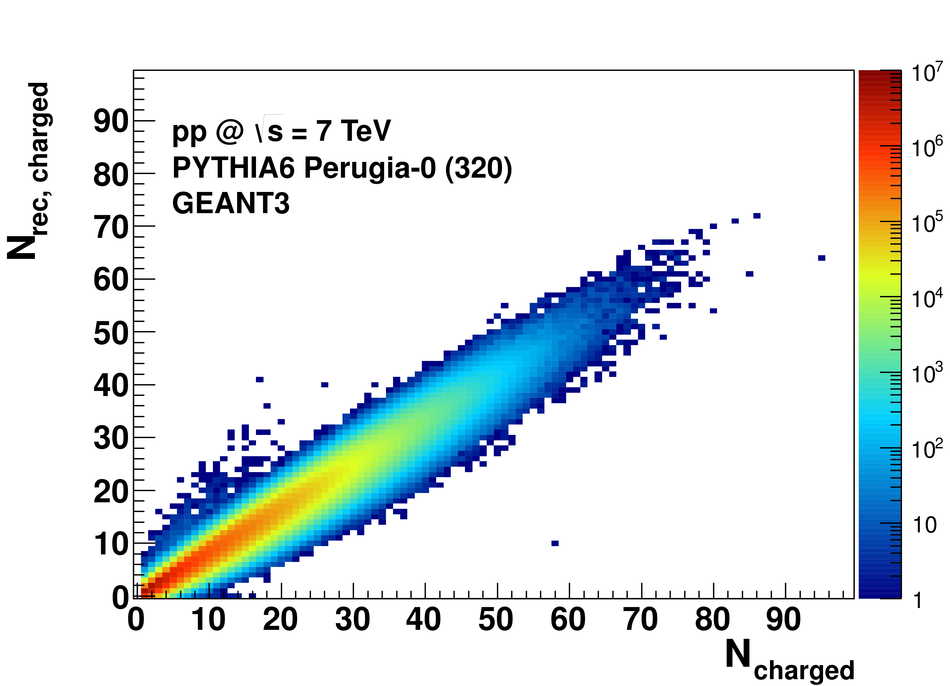## Figure 9

 Azimuthal correlation for events with $\Nch=10$ measured at $\sqrt{s}=7$ TeV.## Figure 10

 Per-trigger near-side pair yield, per-trigger away-side pair yield, per-trigger pair yield in the combinatorial background, average number of trigger particles and average number of uncorrelated seeds measured at $\sqrt{s}=7$ TeV.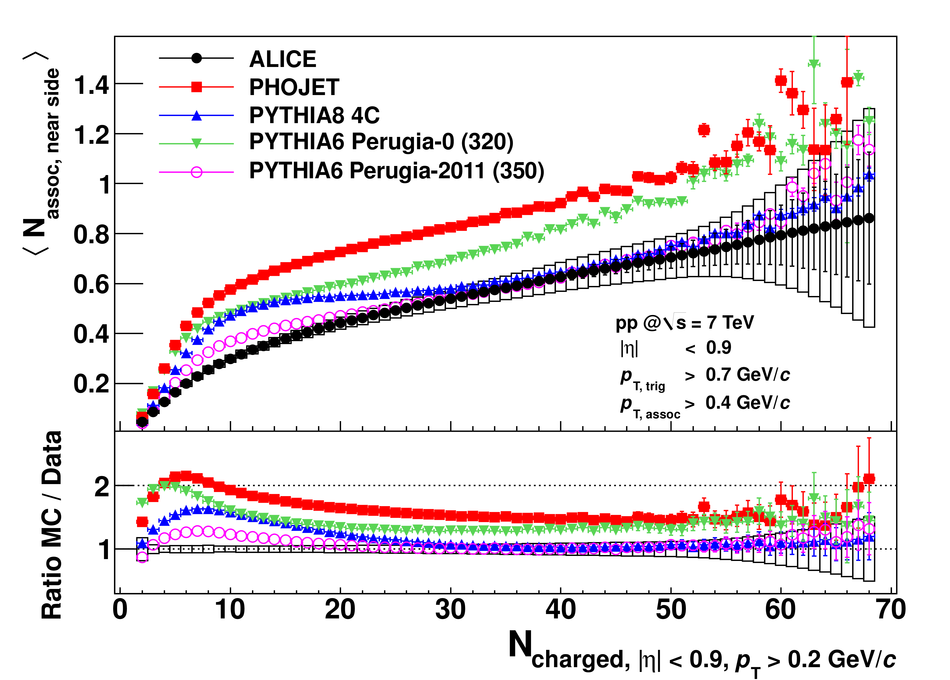## Figure 11

 Per-trigger near-side pair yield, per-trigger away-side pair yield, per-trigger pair yield in the combinatorial background, average number of trigger particles and average number of uncorrelated seeds measured at $\sqrt{s}=2.76$ TeV.## Figure 12

 Per-trigger near-side pair yield, per-trigger away-side pair yield, per-trigger pair yield in the combinatorial background, average number of trigger particles and average number of uncorrelated seeds measured at $\sqrt{s}=0.9$ TeV.## Figure 13

 Per-trigger near-side pair yield as a function of the charged particle multiplicity measured for $\sqrt{s}=0.9$, 2.76, and 7 TeV.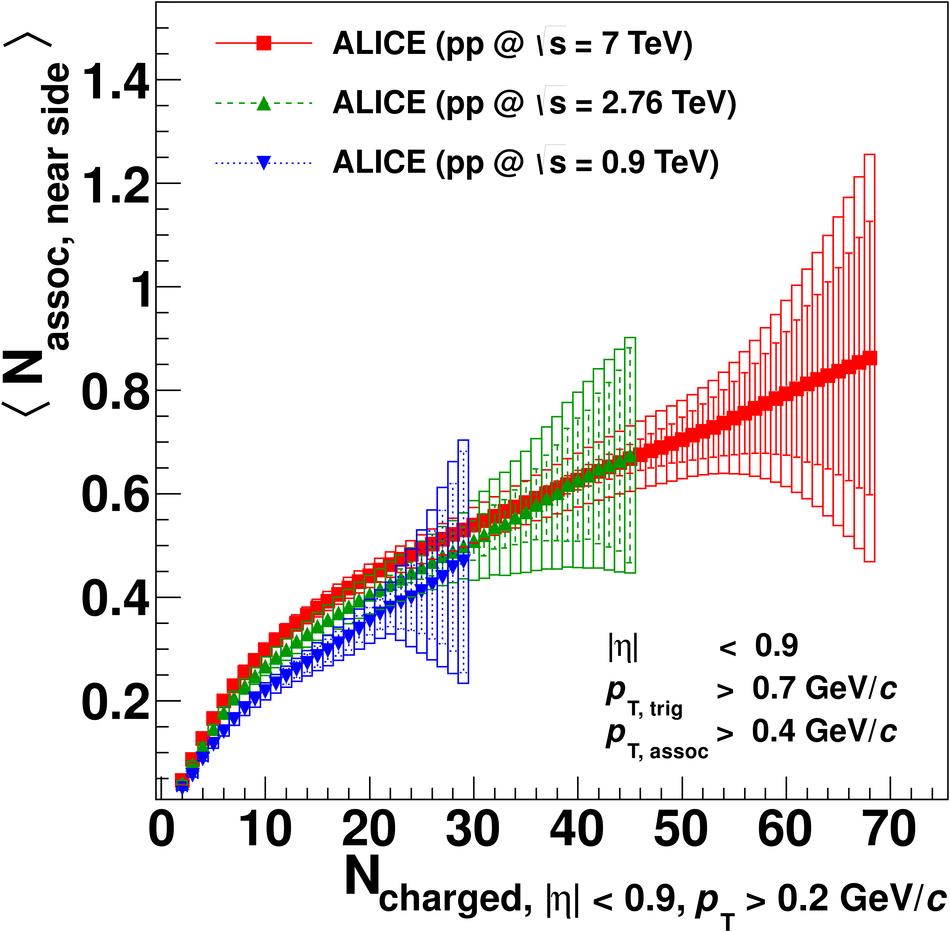## Figure 14

 Per-trigger away-side pair yield as a function of the charged particle multiplicity measured for $\sqrt{s}=0.9$, 2.76, and 7 TeV.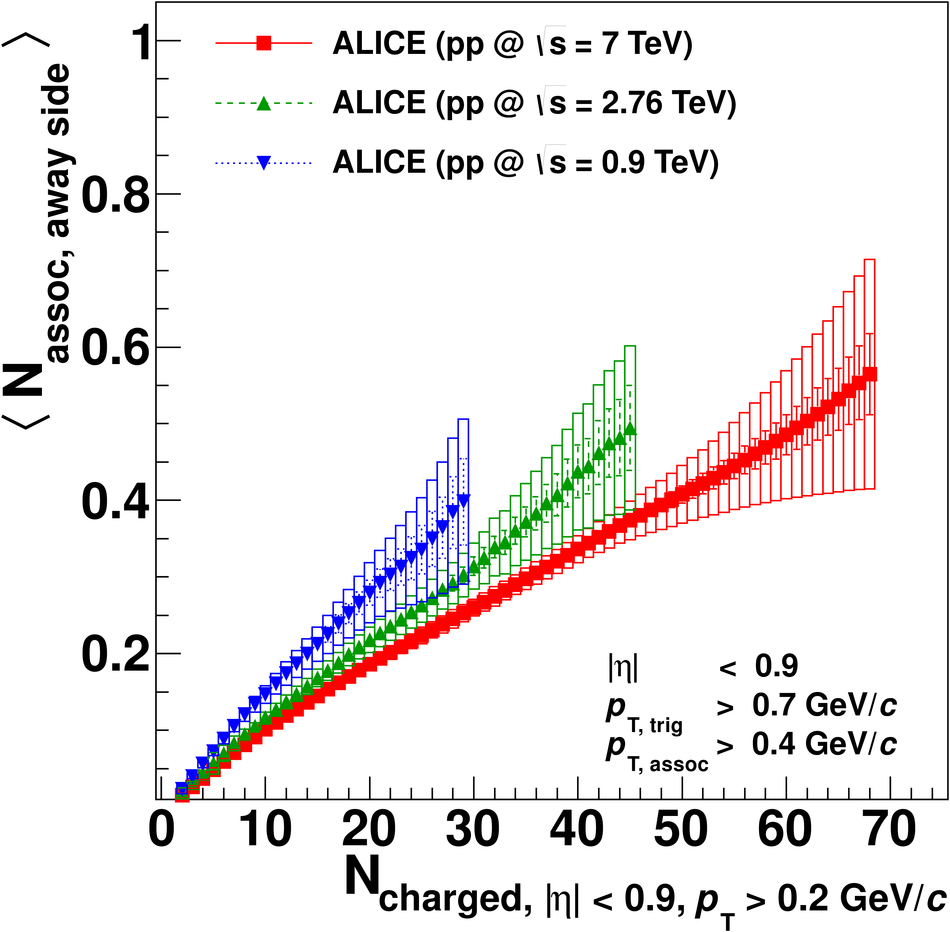## Figure 15

 Per-trigger pair yield in the combinatorial background as a function of the charged particle multiplicity measured for $\sqrt{s}=0.9$, 2.76, and 7 TeV.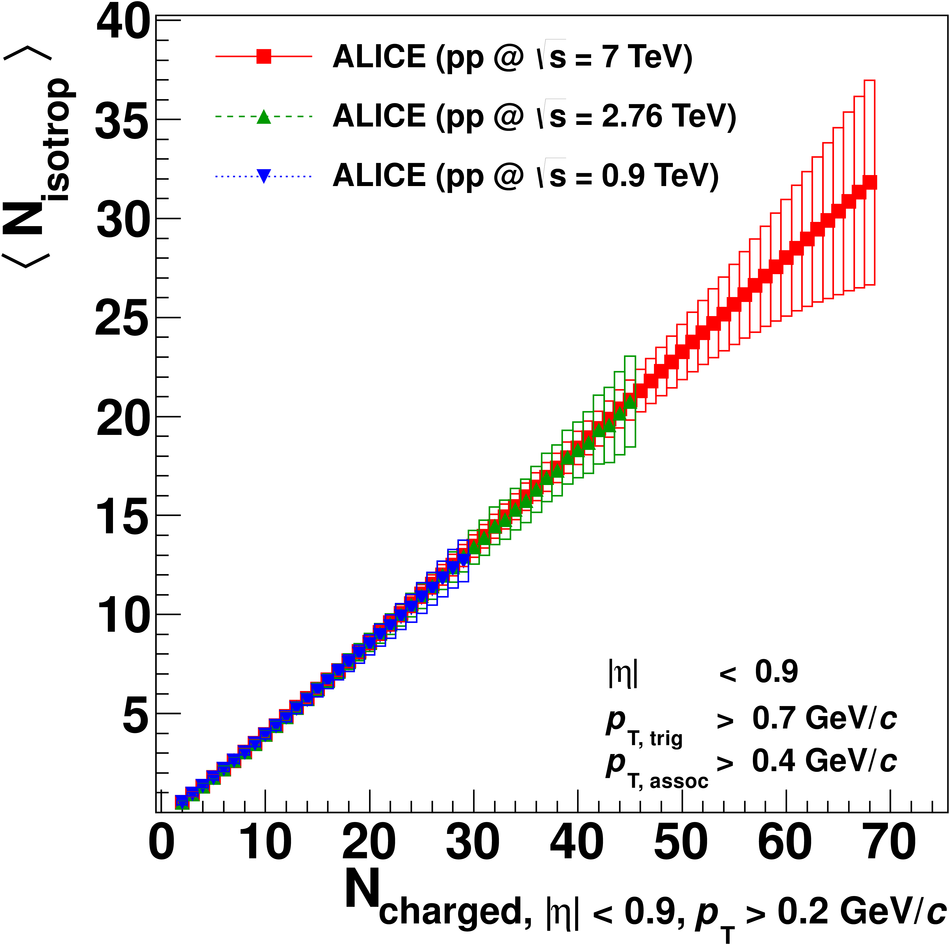## Figure 16

 Average number of trigger particles per event as a function of the charged particle multiplicity measured for $\sqrt{s}=0.9$, 2.76, and 7 TeV.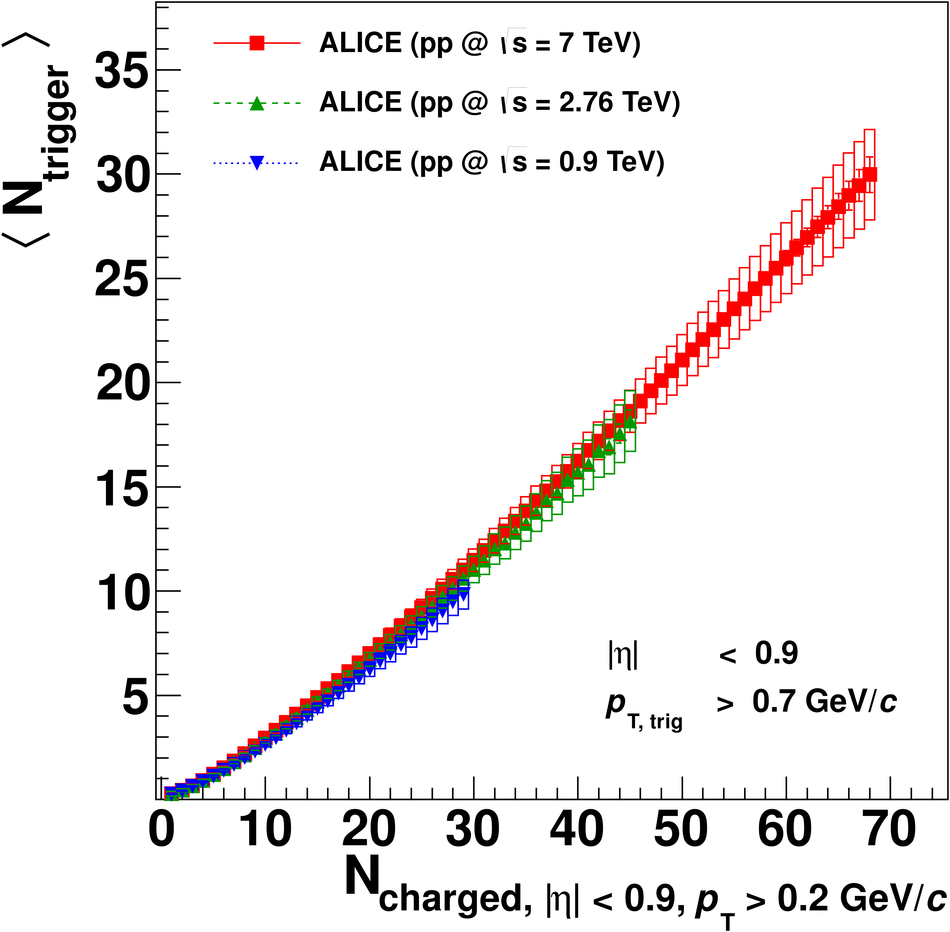## Figure 17

 Average number of uncorrelated seeds per event as a function of the charged particle multiplicity measured for $\sqrt{s}=0.9$, 2.76, and 7 TeV.## Figure 18

 Residual between the number of uncorrelated seeds and linear fit functions.